### Substitution models and distance estimation

When estimating the evolutionary distance between organisms, one needs a model of how frequently different mutations occur in the DNA. Such models are known as substitution models. Our Model Testing and Maximum Likelihood Phylogeny tools currently support the five nucleotide substitution models listed here:

Common to all these models is that they assume mutations at different sites in the genome occur independently and that the mutations at each site follow the same common probability distribution. Thus all five models provide relative frequencies for each of the 16 possible DNA substitutions (e.g.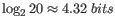,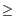,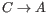,...).

The Jukes-Cantor and Kimura 80 models assume equal base frequencies and the HKY and GTR models allow the frequencies of the four bases to differ (they will be estimated by the observed frequencies of the bases in the alignment). In the Jukes-Cantor model all substitutions are assumed to occur at equal rates, in the Kimura 80 and HKY models transition and transversion rates are allowed to differ (substitution between two purines (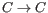) or two pyrimidines (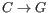) are transitions and purine - pyrimidine substitutions are transversions). The GTR model is the general time reversible model that allows all substitutions to occur at different rates. For the substitution rate matrices describing the substitution models we use the parametrization of Yang [Yang, 1994a].

For protein sequences, our Maximum Likelihood Phylogeny tool supports four substitution models:

As with nucleotide substitution models, it is assumed that mutations at different sites in the genome occur independently and according to the same probability distribution.

The Bishop-Friday model assumes all amino acids occur with same frequency and that all substitutions are equally likely. This is the simplest model, but also the most unrealistic. The remaining three models use amino acid frequencies and substitution rates which have been determined from large scale experiments where huge sets of protein sequences have been aligned and rates have been estimated. These three models reflect the outcome of three different experiments. We recommend using WAG as these rates where estimated from the largest experiment.